JEE  >  Subjective Type Questions: Electrochemistry- 2 | JEE Advanced

# Subjective Type Questions: Electrochemistry- 2 | JEE Advanced - Notes | Study Chemistry 35 Years JEE Main & Advanced Past year Papers - JEE

 1 Crore+ students have signed up on EduRev. Have you?

15. Calculate the quantity of electricity that would be required to reduce 12.3 g of nitrobenzene to aniline, if the current efficiency for the process is 50 per cent. If the potential drop across the cell is 3.0 volts, how much energy will be consumed?                 (1990 - 3 Marks)

Ans:  115800 C, 347.4 kJ

Solution: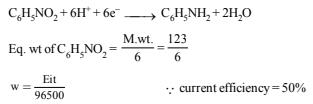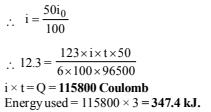16. Zinc granules are added in excess to a 500 ml. of 1.0 M nickel nitrate solution at 25ºC until the equilibrium is reached. If the standard reduction potential of Zn2+ | Zn and Ni2+ | Ni are –0.75 V and –0.24 V respectively, find out the concentration of Ni2+ in solution at equilibrium.                   (1991 - 2 Marks)

Ans:  5.128´10-18 mol l-1

Solution: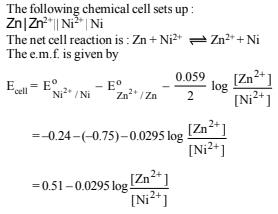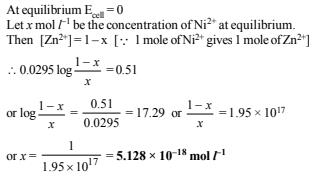17. A current of 1.70 A is passed through 300.0 ml of 0.160 M solution of a ZnSO4 for 230 sec. with a current efficiency of 90%. Find out the molarity of Zn2+ after the deposition of Zn. Assume the volume of the solution to remain constant during the electrolysis.             (1991 - 4 Marks)

Ans:  0.154 M

Solution: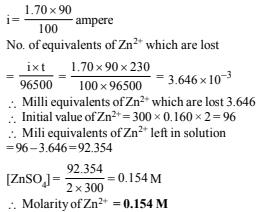18. For the galvanic cell.                                 (1992 - 4 Marks)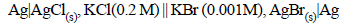Calculate the EMF generated and assign correct polarity to each electrode for a spontaneous process after taking into account the cell reaction at 25ºC.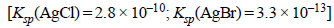Ans:  – 0.037 V

Solution: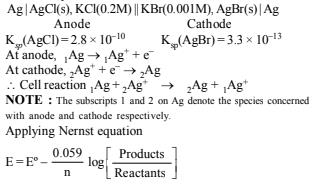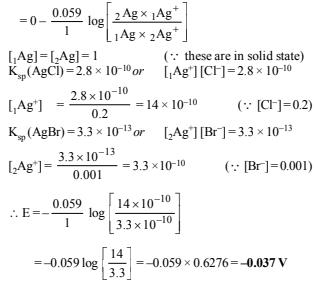Since emf is negative this shows that the reaction is non- spontaneous.

NOTE: For the reaction to be spontaneous, its emf should be positive i.e. E = 0.037 V and its polarities should be reversed i.e. anode should be made cathode and vice-versa. So the galvanic cell is : Ag | AgBr(s), KBr || AgCl(s), KCl | Ag In other words, Ag | AgBr acts as anode and AgCl | Ag acts as cathode.

19. An aqueous solution of NaCl on electrolysis gives H2(g), Cl2(g) and NaOH according to the reaction :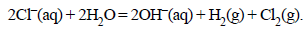A direct current of 25 amperes with a current efficiency of 62% is passed through 20 litres of NaCl solution (20% by weight). Write down the reactions taking place at the anode and the cathode. How long will it take to produce 1 kg of Cl2? What will be the molarity of the solution with respect to hydroxide ion? (Assume no loss due to evaporation.)                       (1992 - 3 Marks)

Ans:  48.69 hrs., 1.408 M

Solution: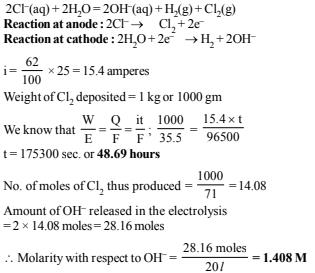20.The standard reduction potential for the half-cell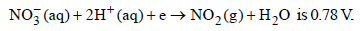(i) Calculate the reduction potential in 8 M H+
(ii) What will be the reduction potential of the half-cell in a  neutral solution? Assume all the other species to be at  unit concentration. (1993 - 2 Marks)

Ans:  (i) 0.887 V, (ii) 0.046 V

Solution: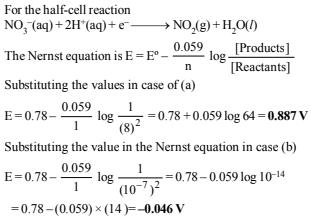21. Chromium metal can be plated out from an acidic solution  containing CrO3 according to the following equation.
CrO3(aq) 6H (aq) 6e →Cr(s) 3H2O
Calculate (i) how many grams of chromium will be plated out  by 24,000 coulombs and (ii) how long will it take to plate out  1.5 g of chromium by using 12.5 amp current.                        (1993 - 2 Marks)

Ans: (i) 2.1554 g of Cr, (ii) 1336.15 sec

Solution: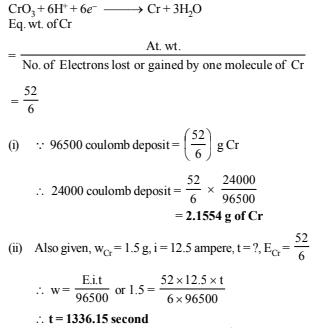22. The standard reduction potential of the Ag+ /Ag electrode at 298 K is 0.799 V. Given that for AgI, Ksp = 8.7 × 10–17, evaluate the potential of the Ag+/Ag electrode in a saturated solution of AgI. Also calculate the standard reduction potential of the I/ AgI/Ag electrode.           (1994 - 3 Marks)

Ans:  0.325 V, – 0.149 V

Solution :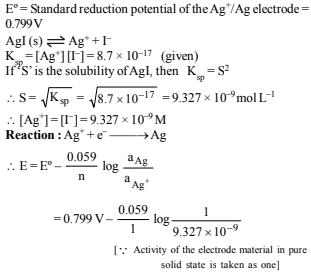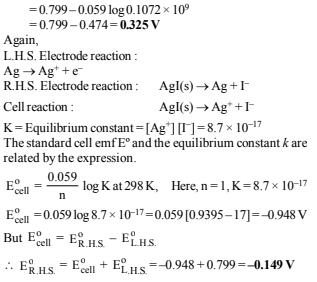23. The Edison storage cells is represented as
Fe(s) | FeO(s) | KOH(aq) | Ni2O3(s) | Ni(s)
The half-cell reactions are :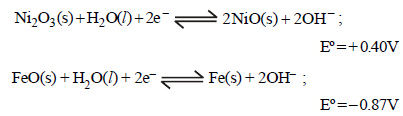(i) What is the cell reaction ?
(ii) What is the cell e.m.f ? How does it depend on the concentration of KOH?
(iii) What is the maximum amount of electrical energy that can be obtained from one mole of Ni2O3?                               (1994 - 4 Marks)

Ans: (ii) 1.27 V, (iii) 2.45×105J

Solution :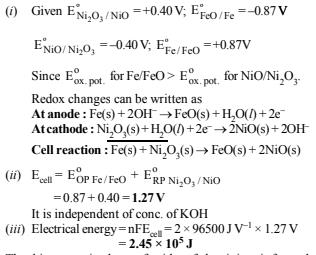24. Although aluminium is above hydrogen in the electrochemical series, it is stable in air and water. Explain.              (1994 - 1 Mark)

Solution :

The thin protective layer of oxides of aluminium is formed which protects the metal from further attack of water and air and make it stable.

25. An excess of liquid mercury is added to an acidified solution of 1.0 × 10–3 M Fe3+. It is found that 5% of Fe3+ remains at equilibrium at 25°C. Calculate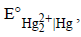assuming that the only reaction that occurs is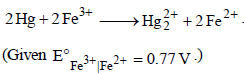(1995 - 4 Marks)

Ans:  0.792 V

Solution :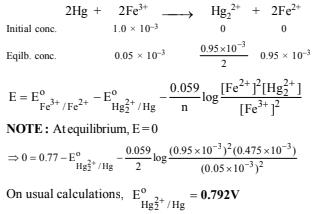26. The standard reduction potential for Cu2+| Cu is +0.34 V. Calculate the reduction potential at pH = 14 for the above couple. Ksp of Cu(OH)2 is 1.0×10–19 (1996 - 3 Marks)

Ans:  – 0.22 V

Solution :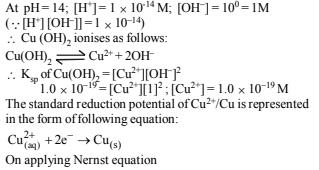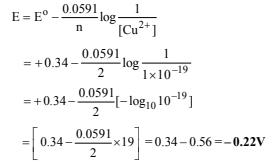The document Subjective Type Questions: Electrochemistry- 2 | JEE Advanced - Notes | Study Chemistry 35 Years JEE Main & Advanced Past year Papers - JEE is a part of the JEE Course Chemistry 35 Years JEE Main & Advanced Past year Papers.
All you need of JEE at this link: JEE

## Chemistry 35 Years JEE Main & Advanced Past year Papers

142 docs|66 tests
 Use Code STAYHOME200 and get INR 200 additional OFF

## Chemistry 35 Years JEE Main & Advanced Past year Papers

142 docs|66 tests

Track your progress, build streaks, highlight & save important lessons and more!

,

,

,

,

,

,

,

,

,

,

,

,

,

,

,

,

,

,

,

,

,

;